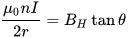# Finding the Earth's magnetic field strength using a Galvanometer

## Homework Statement

[/B]
I am working on a experiment on finding the Earth's magnetic field strength in Denmark. Using a Galvanometer and a constant power source of 5.1V and slowly adjusting the resistance, I was able to find the changes in angle in relation to the changes of current.

2. Homework Equations

https://en.wikipedia.org/wiki/Galvanometer#TheoryThen I used the equation to find the Bh, the horizontal component BH of the Earth's magnetic field.

## The Attempt at a Solution

However, my calculation shows my Bh is 5.62E-04, while the actual Bh is 5.03E-05. There is a difference of factor of 10!!! I went through the calculations with my teacher, but she could not find the problem. I am hoping that someone can help me locating the error in the experiment/calculation.

I would really appreciate any help! Thank you!

#### Attachments

Homework Helper
Gold Member
I presume the wire you used was insulated, so that the path of the current was through the path of the wire, and not some shortcut. 50 turns with I=200 mA should have caused a much bigger deflection in the compass needle than what you got. ## \\ ## Meanwhile the currents you are reading are inconsistent with the voltage source and the size of the series resistance. It appears to me that most likely your current readings are about a factor of 10 too high, and that your series resistance readings are also incorrect. ## \\ ## See also: https://www.ngdc.noaa.gov/geomag-web/#igrfwmm Using latitude =56 N and longitude 9 E,(approximate location of Denmark) the program gives the horizontal component as ## B_H=.17 \cdot 10^{-4} ## T, compared with your (I believe inaccurate )## B_H=.503 \cdot 10^{-4} ## T. This puts your values off by a factor of 30 rather than 10.

Last edited:
haruspex
Homework Helper
Gold Member
## B_H=.17 \cdot 10^{-4} ## T, compared with your (I believe inaccurate )## B_H=.503 \cdot 10^{-4} ## T. This puts your values off by a factor of 30 rather than 10.
That looks like 3:1 rather than 30:1. Am I missing something? I haven't read the rest of the thread.

Thank you for the link! For Copenhagen, I get the following components.

Copenhagan, Denmark
Latitude: 55° 40' 0" N
Longitude: 12° 34' 60" E

Horizontal Intensity = 17,124.7 nT
North Comp = 17,086.8 nT
East Comp = 1,139.1 nT
Vertical Comp = 47,328.6 nT
Total field = 50,331.4 nT

I assume the horizontal intensity is the Bh 17,124.7 nT or 0.17 E-4 T. My inaccurate value is
5.62E-04 T, which is indeed off by a factor of 30.

Last edited:
Homework Helper
Gold Member
Thank you for the link! For Copenhagen, I get the following components.

Copenhagan, Denmark
Latitude: 55° 40' 0" N
Longitude: 12° 34' 60" E

Horizontal Intensity = 17,124.7 nT
North Comp = 17,086.8 nT
East Comp = 1,139.1 nT
Vertical Comp = 47,328.6 nT
Total field = 50,331.4 nT

I assume the horizontal intensity is the Bh 17,124.7 nT or 0.17 E-4 nT. My inaccurate value is 0.503 E-5 nT, which is indeed off by a factor of 30.
Your 5.62 E-04 T is the one that is off by a factor of 30. I would have to believe the problem may be with your current meter, unless you used bare wire instead of insulated wire.

Homework Helper
Gold Member
That looks like 3:1 rather than 30:1. Am I missing something? I haven't read the rest of the thread.
The experimental value that the OP got is ## B_H=## 5.62 E-04 T.

andrevdh
Homework Helper
In the theory section it advises you to adjust the current for a 45o deflection of the galvanometer and use that value to calculate BH

•Homework Helper
Gold Member
In the theory section it advises you to adjust the current for a 45o deflection of the galvanometer and use that value to calculate BH
The ## \theta=10 ## degrees is a pretty good size. That along with the reasonably good straight line of the data would indicate that there was some consistency. There is a gross error somewhere though that the OP has yet to locate. One possible cause is that the wire may have been bare and non-insulated=the OP has yet to respond to my question on that item. The OP would have needed nearly 1.0 amps of current with 50 turns to get a 45 degree deflection, based on the readings at lower current levels. There is something very incorrect with the numbers he obtained. 50 turns with 1.0 amps would produce a very sizable magnetic field=much stronger than the earth's magnetic field. ## \\ ## One additional question also worth asking: Was the set of loops in a vertical plane so the magnetic field from the current was perpendicular the compass needle, as opposed to being in a horizontal plane? They provided pictures in the "link" the OP provided, so hopefully that is not the problem.

Last edited:
The experimental value that the OP got is ## B_H=## 5.62 E-04 T.

What is "OP"?:-)

Homework Helper
Gold Member
What is "OP"?:-)
Original poster and/or original post.

andrevdh
Homework Helper
Maybe the plane of the coil was not aligned with magnetic north or something in the vicinity has offset the earth's magnetic field?

Hi all, I don't think I have the chance to do another experiment. The experiment was done on my teacher desk which has a lot of electronics and power supply below the desk. I am looking into all the suggestions of possible causes to bad result. Thank you for the feedback. I really appreciate them!

•